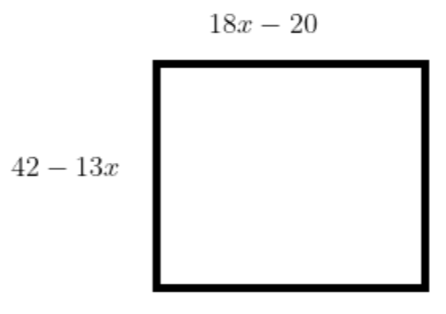QuestionAnswers

# If one side of a square is represented by $18x-20$ and the adjacent sides are represented by $42-13x$, find the area of the square.Verified
129k+ views
Hint: In general a square is a parallelogram with all sides equal, adjacent sides are perpendicular and opposite sides are parallel to each other. Hence first we equate the given side to find the value of x and then we can find the value of the side of the square by using the calculated value of x.

Complete step-by-step solution:
To find the area, we multiply the base and height for a parallelogram, in the case of a square. Both base and height are equal to each other. So we will do $\text{side }\!\!\times\!\!\text{ side}$ .
The given sides of the square are $18x-20$ and $42-13x$. According to the definition of the square, all four sides of the square are equal.So we can equate two sides of a square.
Hence it can be written as
$\Rightarrow 18x-20=42-13x$
On collecting like terms
$\Rightarrow 18x+13x=42+20$
$\Rightarrow 31x=62$
$\Rightarrow x=2$
On substituting $x=2$ in given sides of the square.
At $x=2$, value of $18x-20$ is calculated below
$\Rightarrow 18\times 2-20$
$\Rightarrow 36-20$
$\Rightarrow 16$
At $x=2$, value of $42-13x$
$\Rightarrow 42-13\times 2$
$\Rightarrow 42-26$
$\Rightarrow 16$
If the side of the square is a then area(A) of the square is
$\Rightarrow A=a\times a$
$\Rightarrow A={{a}^{2}}$
In the given question the side of the square is 16.
Required area is
$\Rightarrow A={{\left( 16 \right)}^{2}}$
$\Rightarrow A=256\,\text{square}\,\text{units}$.

Note: As after solving the equation we will get the value of side a but in question, we need to find the value of the area of the square. Hence after getting the value of the side of the square, don’t forget to find the area of the square by multiplying the sides.